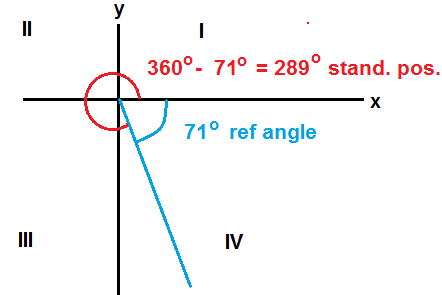# The tutor talks more about how to find the angle in standard position from the reference angle.

Let’s imagine you know the reference angle is 71°, but the actual angle is in QIV. The reference angle always extends from the x-axis into the quadrant of interest. From the illustration you can see the way to the angle in standard position:In each case, the reference angle should be drawn in its quadrant. From the drawing it’s then obvious whether to add or subtract the reference angle, and to or from what number, in order to find the angle in standard position. However, here is a guide of how to arrive at the angle in standard position from a reference angle:

QII: 180° – ref angle.

QIII: 180° + ref angle.

QIV: 360 – ref angle.

HTH:)

Source:

Travers, Kenneth J. et al. Using Advanced Algebra. Toronto: Doubleday, 1977.

Jack of Oracle Tutoring by Jack and Diane, Campbell River, BC.